Add penalties to a conservation planning problem() to account for symmetric connectivity between planning units. Symmetric connectivity data describe connectivity information that is not directional. For example, symmetric connectivity data could describe which planning units are adjacent to each other (see adjacency_matrix(), or which planning units are within threshold distance of each other (see proximity_matrix()).

# S4 method for ConservationProblem,ANY,ANY,matrix

# S4 method for ConservationProblem,ANY,ANY,Matrix

# S4 method for ConservationProblem,ANY,ANY,data.frame

# S4 method for ConservationProblem,ANY,ANY,dgCMatrix

# S4 method for ConservationProblem,ANY,ANY,array
add_connectivity_penalties(x, penalty, zones, data)

## Arguments

x

problem() (i.e., ConservationProblem) object.

penalty

numeric penalty that is used to scale the importance of selecting planning units with strong connectivity between them compared to the main problem objective (e.g., solution cost when the argument to x has a minimum set objective set using add_min_set_objective()). Higher penalty values can be used to obtain solutions with a high degree of connectivity, and smaller penalty values can be used to obtain solutions with a small degree of connectivity. Note that negative penalty values can be used to obtain solutions that have very little connectivity.

zones

matrix or Matrix object describing the level of connectivity between different zones. Each row and column corresponds to a different zone in the argument to x, and cell values indicate the level of connectivity between each combination of zones. Cell values along the diagonal of the matrix represent the level of connectivity between planning units allocated to the same zone. Cell values must lay between 1 and -1, where negative values favor solutions with weak connectivity. The default argument to zones is an identity matrix (i.e., a matrix with ones along the matrix diagonal and zeros elsewhere), so that planning units are only considered to be connected when they are allocated to the same zone. This argument is required when working with multiple zones and the argument to data is a matrix or Matrix object. If the argument to data is an array or data.frame with data for multiple zones (e.g., using the "zone1" and "zone2" column names), this argument must explicitly be set to NULL otherwise an error will be thrown.

data

matrix, Matrix, data.frame, or array object containing connectivity data. The connectivity values correspond to the strength of connectivity between different planning units. Thus connections between planning units that are associated with higher values are more favorable in the solution. See the Data format section for more information.

## Value

Object (i.e., ConservationProblem) with the penalties added to it.

## Details

This function adds penalties to conservation planning problem to penalize solutions that have low connectivity. Specifically, it favors pair-wise connections between planning units that have high connectivity values (based on Önal and Briers 2002).

## Data format

The argument to data can be specified using several different formats.

data as a matrix/Matrix object

where rows and columns represent different planning units and the value of each cell represents the strength of connectivity between two different planning units. Cells that occur along the matrix diagonal are treated as weights which indicate that planning units are more desirable in the solution. The argument to zones can be used to control the strength of connectivity between planning units in different zones. The default argument for zones is to treat planning units allocated to different zones as having zero connectivity.

data as a data.frame object

containing the fields (columns) "id1", "id2", and "boundary". Here, each row denotes the connectivity between a pair of planning units (per values in the "id1" and "id2" columns) following the Marxan format. If the argument to x contains multiple zones, then the columns "zone1" and "zone2" can optionally be provided to manually specify the connectivity values between planning units when they are allocated to specific zones. If the columns "zone1" and "zone2" are present, then the argument to zones must be NULL.

data as an array object

containing four-dimensions where cell values indicate the strength of connectivity between planning units when they are assigned to specific management zones. The first two dimensions (i.e., rows and columns) indicate the strength of connectivity between different planning units and the second two dimensions indicate the different management zones. Thus the data[1, 2, 3, 4] indicates the strength of connectivity between planning unit 1 and planning unit 2 when planning unit 1 is assigned to zone 3 and planning unit 2 is assigned to zone 4.

## Mathematical formulation

The connectivity penalties are implemented using the following equations. Let $$I$$ represent the set of planning units (indexed by $$i$$ or $$j$$), $$Z$$ represent the set of management zones (indexed by $$z$$ or $$y$$), and $$X_{iz}$$ represent the decision variable for planning unit $$i$$ for in zone $$z$$ (e.g., with binary values one indicating if planning unit is allocated or not). Also, let $$p$$ represent the argument to penalty, $$D$$ represent the argument to data, and $$W$$ represent the argument to zones.

If the argument to data is supplied as a matrix or Matrix object, then the penalties are calculated as:

$$\sum_{i}^{I} \sum_{j}^{I} \sum_{z}^{Z} \sum_{y}^{Z} (-p \times X_{iz} \times X_{jy} \times D_{ij} \times W_{zy})$$

Otherwise, if the argument to data is supplied as a data.frame or array object, then the penalties are calculated as:

$$\sum_{i}^{I} \sum_{j}^{I} \sum_{z}^{Z} \sum_{y}^{Z} (-p \times X_{iz} \times X_{jy} \times D_{ijzy})$$

Note that when the problem objective is to maximize some measure of benefit and not minimize some measure of cost, the term $$-p$$ is replaced with $$p$$.

See penalties for an overview of all functions for adding penalties. Additionally, see add_asym_connectivity_penalties() to account for asymmetric connectivity between planning units.

Other penalties: add_asym_connectivity_penalties(), add_boundary_penalties(), add_feature_weights(), add_linear_penalties()

## Examples

# set seed for reproducibility
set.seed(600)

# load Matrix package for visualizing matrices
require(Matrix)

data(sim_pu_polygons, sim_pu_zones_stack, sim_features, sim_features_zones)

# define function to rescale values between zero and one so that we
# can compare solutions from different connectivity matrices
rescale <- function(x, to = c(0, 1), from = range(x, na.rm = TRUE)) {
(x - from) / diff(from) * diff(to) + to
}

# create basic problem
p1 <- problem(sim_pu_polygons, sim_features, "cost") %>%

# create a symmetric connectivity matrix where the connectivity between
# two planning units corresponds to their shared boundary length
b_matrix <- boundary_matrix(sim_pu_polygons)

# standardize matrix values to lay between zero and one
b_matrix[] <- rescale(b_matrix[])

# visualize connectivity matrix
# \dontrun{
image(b_matrix)# }
# create a symmetric connectivity matrix where the connectivity between
# two planning units corresponds to their spatial proximity
# i.e., planning units that are further apart share less connectivity
centroids <- rgeos::gCentroid(sim_pu_polygons, byid = TRUE)
d_matrix <- (1 / (as(dist(centroids@coords), "Matrix") + 1))

# standardize matrix values to lay between zero and one
d_matrix[] <- rescale(d_matrix[])

# remove connections between planning units without connectivity to
# reduce run-time
d_matrix[d_matrix < 0.7] <- 0

# visualize connectivity matrix
# \dontrun{
image(d_matrix)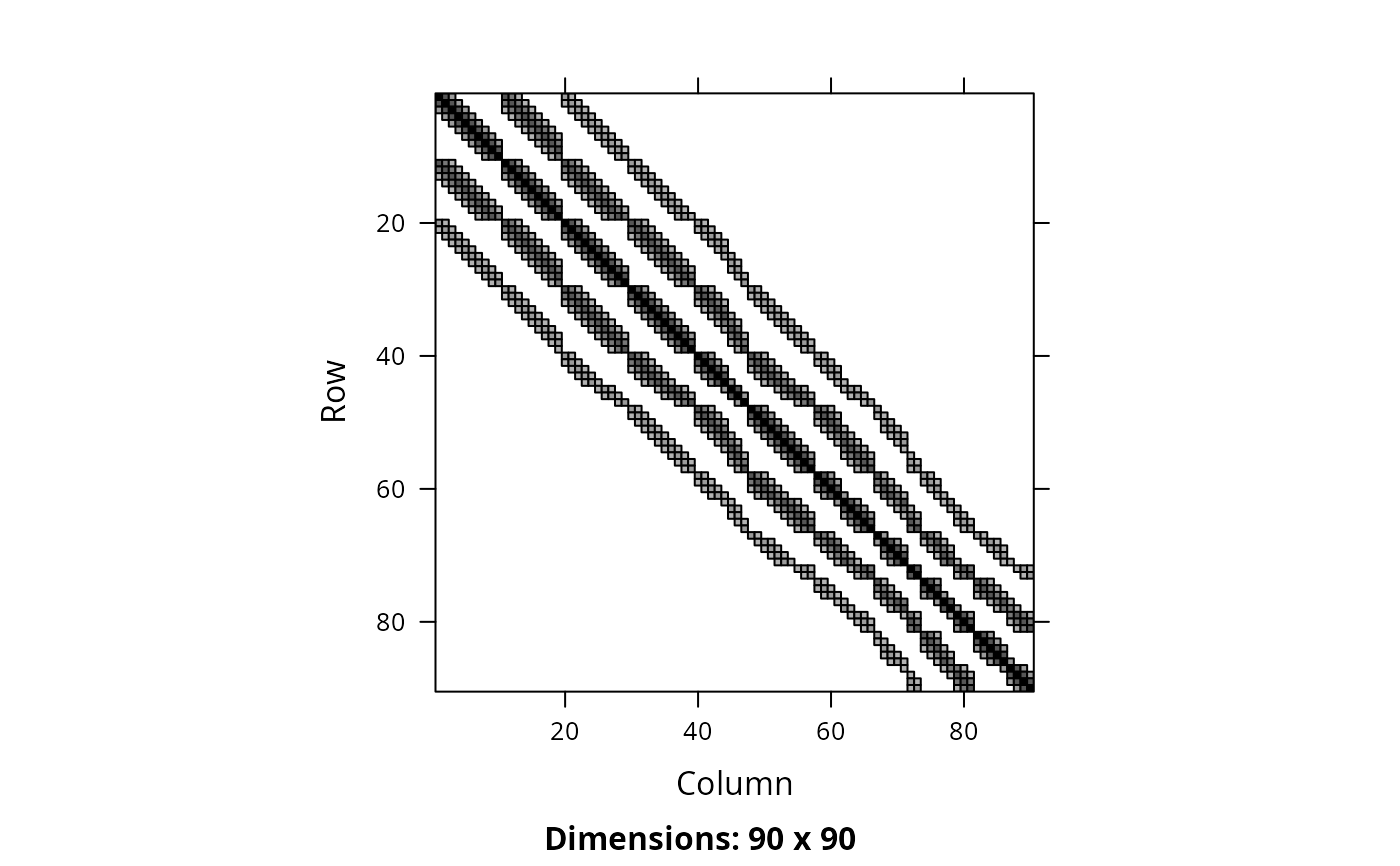# }
# create a symmetric connectivity matrix where the connectivity
# between adjacent two planning units corresponds to their combined
# value in a field in the planning unit attribute data
# for example, this field could describe the extent of native vegetation in
# each planning unit and we could use connectivity penalties to identify
# solutions that cluster planning units together that both contain large
# amounts of native vegetation
c_matrix <- connectivity_matrix(sim_pu_polygons, "cost")

# standardize matrix values to lay between zero and one
c_matrix[] <- rescale(c_matrix[])

# visualize connectivity matrix
# \dontrun{
image(c_matrix)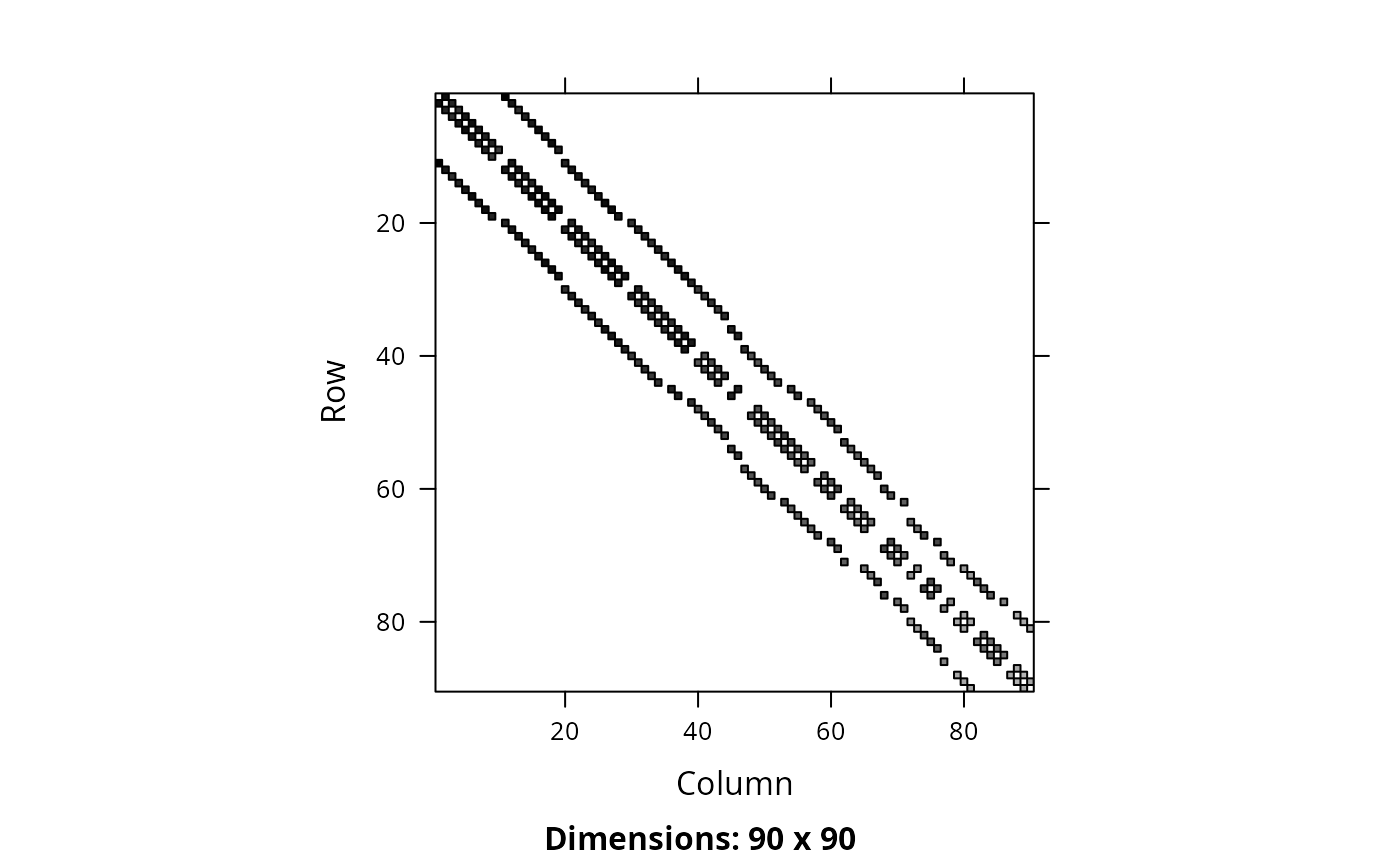# }

# create penalties
penalties <- c(10, 25)

# create problems using the different connectivity matrices and penalties
p2 <- list(p1,
p1 %>% add_connectivity_penalties(penalties, data = b_matrix),
p1 %>% add_connectivity_penalties(penalties, data = b_matrix),
p1 %>% add_connectivity_penalties(penalties, data = d_matrix),
p1 %>% add_connectivity_penalties(penalties, data = d_matrix),
p1 %>% add_connectivity_penalties(penalties, data = c_matrix),
p1 %>% add_connectivity_penalties(penalties, data = c_matrix))

# assign names to the problems
names(p2) <- c("basic problem",
paste0("b_matrix (", penalties,")"),
paste0("d_matrix (", penalties,")"),
paste0("c_matrix (", penalties,")"))
# \dontrun{
# solve problems
s2 <- lapply(p2, solve)

# plot solutions
par(mfrow = c(2, 2))
for (i in seq_along(s2)) {
plot(s2[[i]], main = names(p2)[i], cex = 1.5, col = "white")
plot(s2[[i]][s2[[i]]\$solution_1 == 1, ], col = "darkgreen", add = TRUE)
}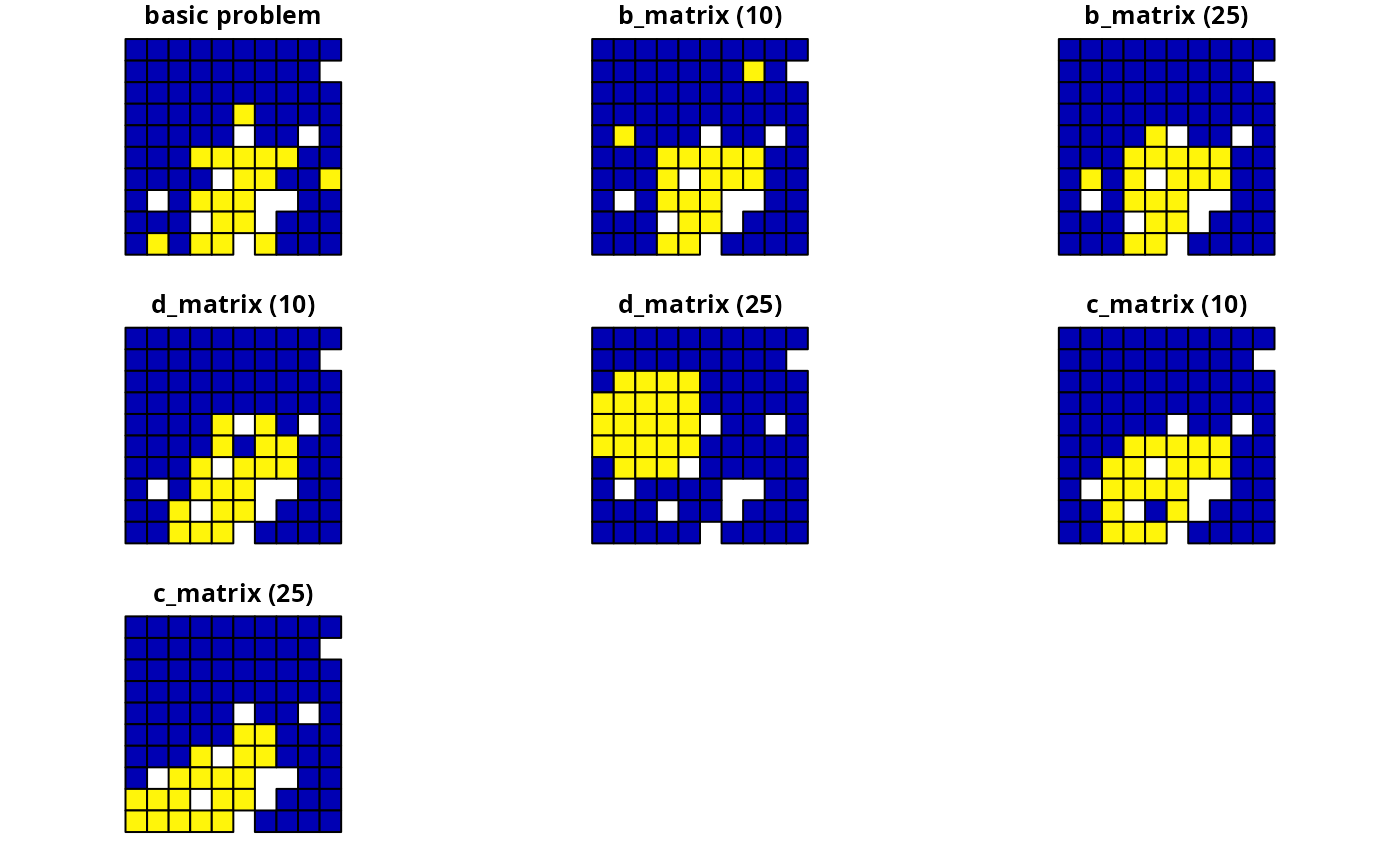# }

# create minimal multi-zone problem and limit solver to one minute
# to obtain solutions in a short period of time
p3 <- problem(sim_pu_zones_stack, sim_features_zones) %>%
add_relative_targets(matrix(0.15, nrow = 5, ncol = 3)) %>%
add_default_solver(time_limit = 60, verbose = FALSE)

# create matrix showing which planning units are adjacent to other units

# visualize matrix
# \dontrun{
image(a_matrix)
# }
# create a zone matrix where connectivities are only present between
# planning units that are allocated to the same zone
zm1 <- as(diag(3), "Matrix")

# print zone matrix
print(zm1)
#>      [,1] [,2] [,3]
#> [1,]    1    .    .
#> [2,]    .    1    .
#> [3,]    .    .    1

# create a zone matrix where connectivities are strongest between
# planning units allocated to different zones
zm2 <- matrix(1, ncol = 3, nrow = 3)
diag(zm2) <- 0
zm2 <- as(zm2, "Matrix")

# print zone matrix
print(zm2)
#> 3 x 3 Matrix of class "dsyMatrix"
#>      [,1] [,2] [,3]
#> [1,]    0    1    1
#> [2,]    1    0    1
#> [3,]    1    1    0

# create a zone matrix that indicates that connectivities between planning
# units assigned to the same zone are much higher than connectivities
# assigned to different zones
zm3 <- matrix(0.1, ncol = 3, nrow = 3)
diag(zm3) <- 1
zm3 <- as(zm3, "Matrix")

# print zone matrix
print(zm3)
#> 3 x 3 Matrix of class "dsyMatrix"
#>      [,1] [,2] [,3]
#> [1,]  1.0  0.1  0.1
#> [2,]  0.1  1.0  0.1
#> [3,]  0.1  0.1  1.0

# create a zone matrix that indicates that connectivities between planning
# units allocated to zone 1 are very high, connectivities between planning
# units allocated to zones 1 and 2 are moderately high, and connectivities
# planning units allocated to other zones are low
zm4 <- matrix(0.1, ncol = 3, nrow = 3)
zm4[1, 1] <- 1
zm4[1, 2] <- 0.5
zm4[2, 1] <- 0.5
zm4 <- as(zm4, "Matrix")

# print zone matrix
print(zm4)
#> 3 x 3 Matrix of class "dsyMatrix"
#>      [,1] [,2] [,3]
#> [1,]  1.0  0.5  0.1
#> [2,]  0.5  0.1  0.1
#> [3,]  0.1  0.1  0.1

# create a zone matrix with strong connectivities between planning units
# allocated to the same zone, moderate connectivities between planning
# unit allocated to zone 1 and zone 2, and negative connectivities between
# planning units allocated to zone 3 and the other two zones
zm5 <- matrix(-1, ncol = 3, nrow = 3)
zm5[1, 2] <- 0.5
zm5[2, 1] <- 0.5
diag(zm5) <- 1
zm5 <- as(zm5, "Matrix")

# print zone matrix
print(zm5)
#> 3 x 3 Matrix of class "dsyMatrix"
#>      [,1] [,2] [,3]
#> [1,]  1.0  0.5   -1
#> [2,]  0.5  1.0   -1
#> [3,] -1.0 -1.0    1

# create vector of penalties to use creating problems
penalties2 <- c(5, 15)

# create multi-zone problems using the adjacent connectivity matrix and
# different zone matrices
p4 <- list(
p3,

# assign names to the problems
names(p4) <- c("basic problem",
paste0("zm", rep(seq_len(5), each = 2), " (",
rep(penalties2, 2), ")"))
# \dontrun{
# solve problems
s4 <- lapply(p4, solve)
s4 <- lapply(s4, category_layer)
s4 <- stack(s4)

# plot solutions
plot(s4, main = names(p4), axes = FALSE, box = FALSE)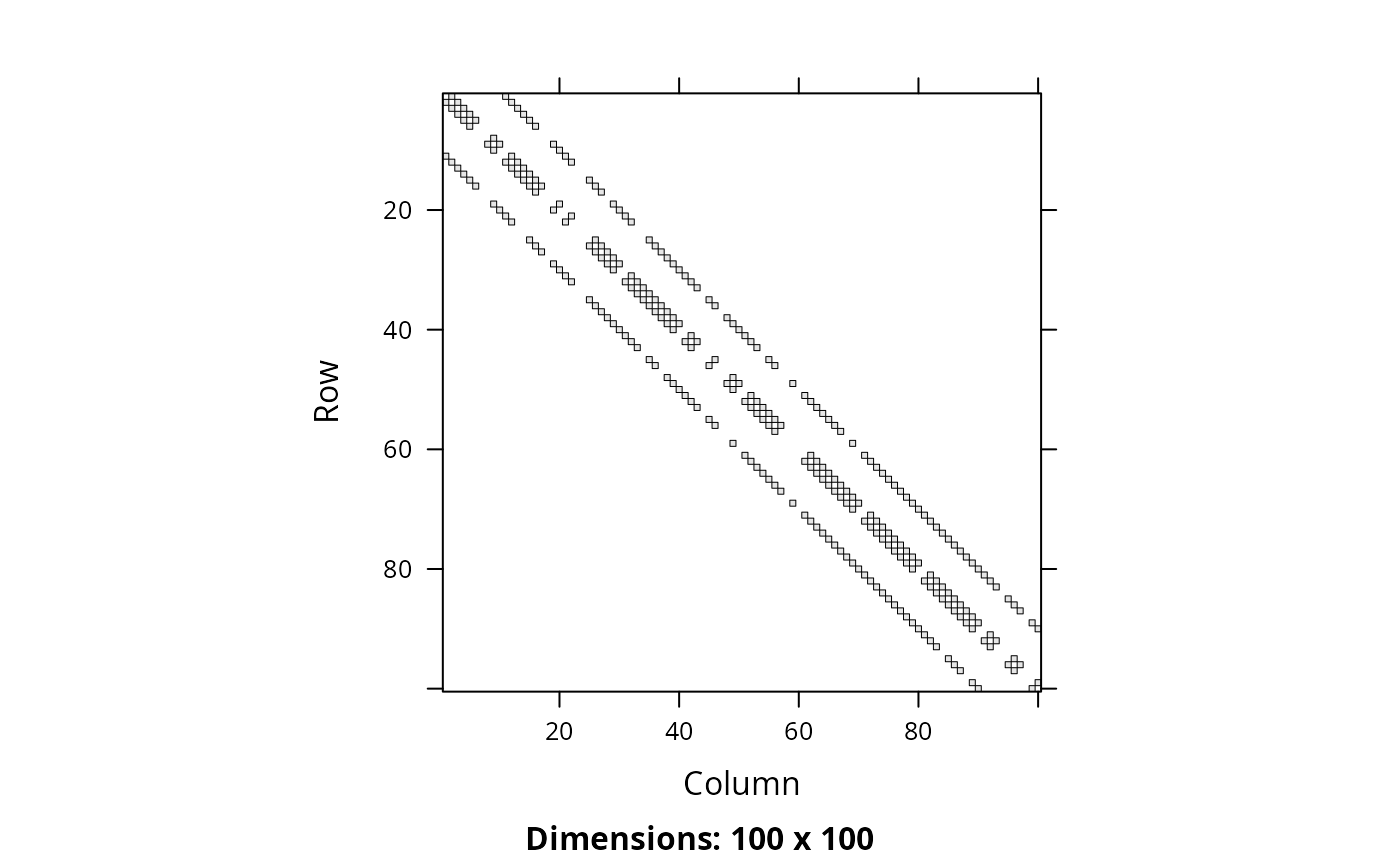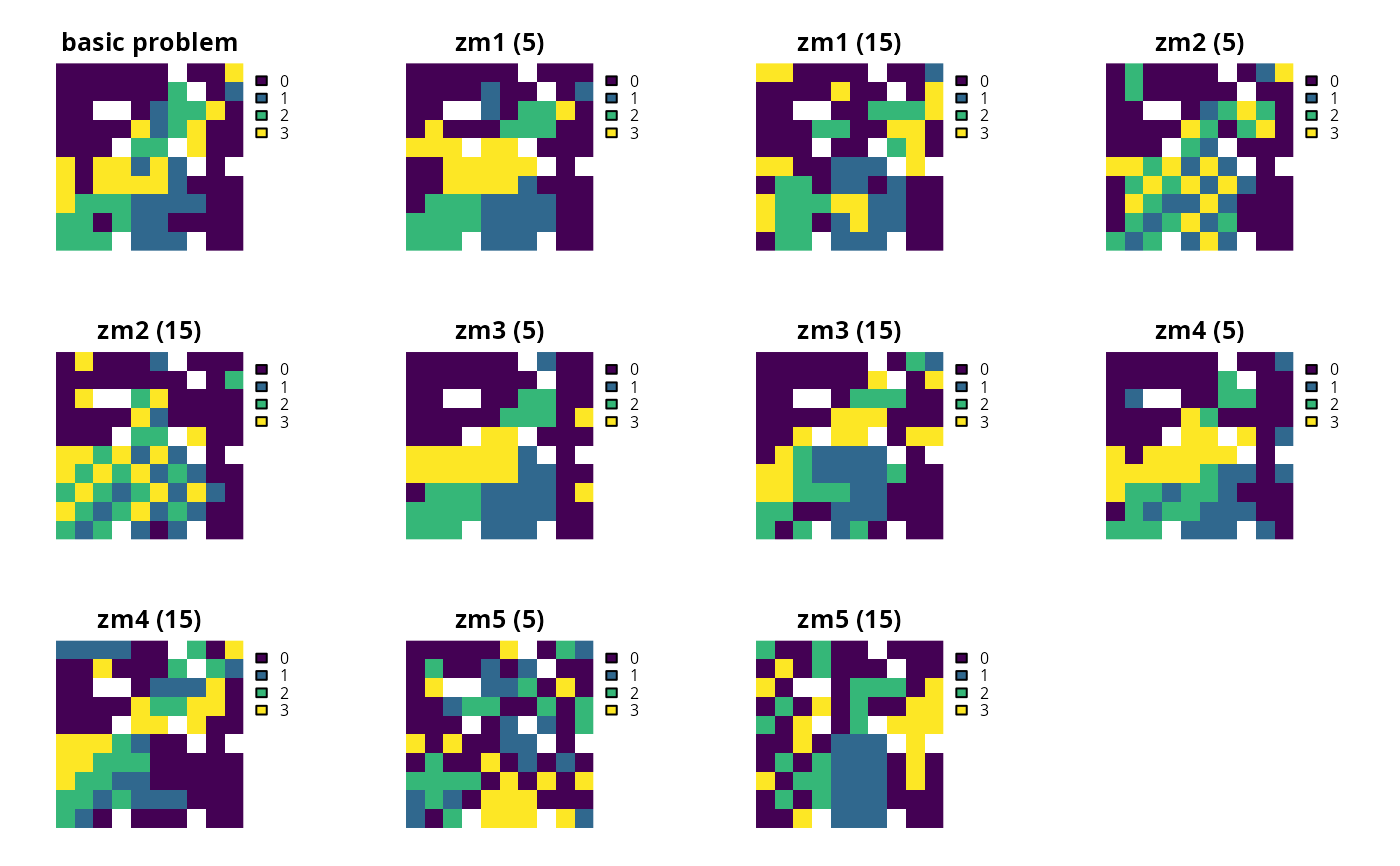# }

# create an array to manually specify the connectivities between
# each planning unit when they are allocated to each different zone
# for real-world problems, these connectivities would be generated using
# data - but here these connectivity values are assigned as random
# ones or zeros
c_array <- array(0, c(rep(ncell(sim_pu_zones_stack[]), 2), 3, 3))
for (z1 in seq_len(3))
for (z2 in seq_len(3))
c_array[, , z1, z2] <- round(runif(ncell(sim_pu_zones_stack[]) ^ 2,
0, 0.505))

# create a problem with the manually specified connectivity array
# note that the zones argument is set to NULL because the connectivity
# data is an array
p5 <- list(p3,
p3 %>% add_connectivity_penalties(15, zones = NULL, c_array))

# assign names to the problems
names(p5) <- c("basic problem", "connectivity array")
# \dontrun{
# solve problems
s5 <- lapply(p5, solve)
s5 <- lapply(s5, category_layer)
s5 <- stack(s5)

# plot solutions
plot(s5, main = names(p5), axes = FALSE, box = FALSE)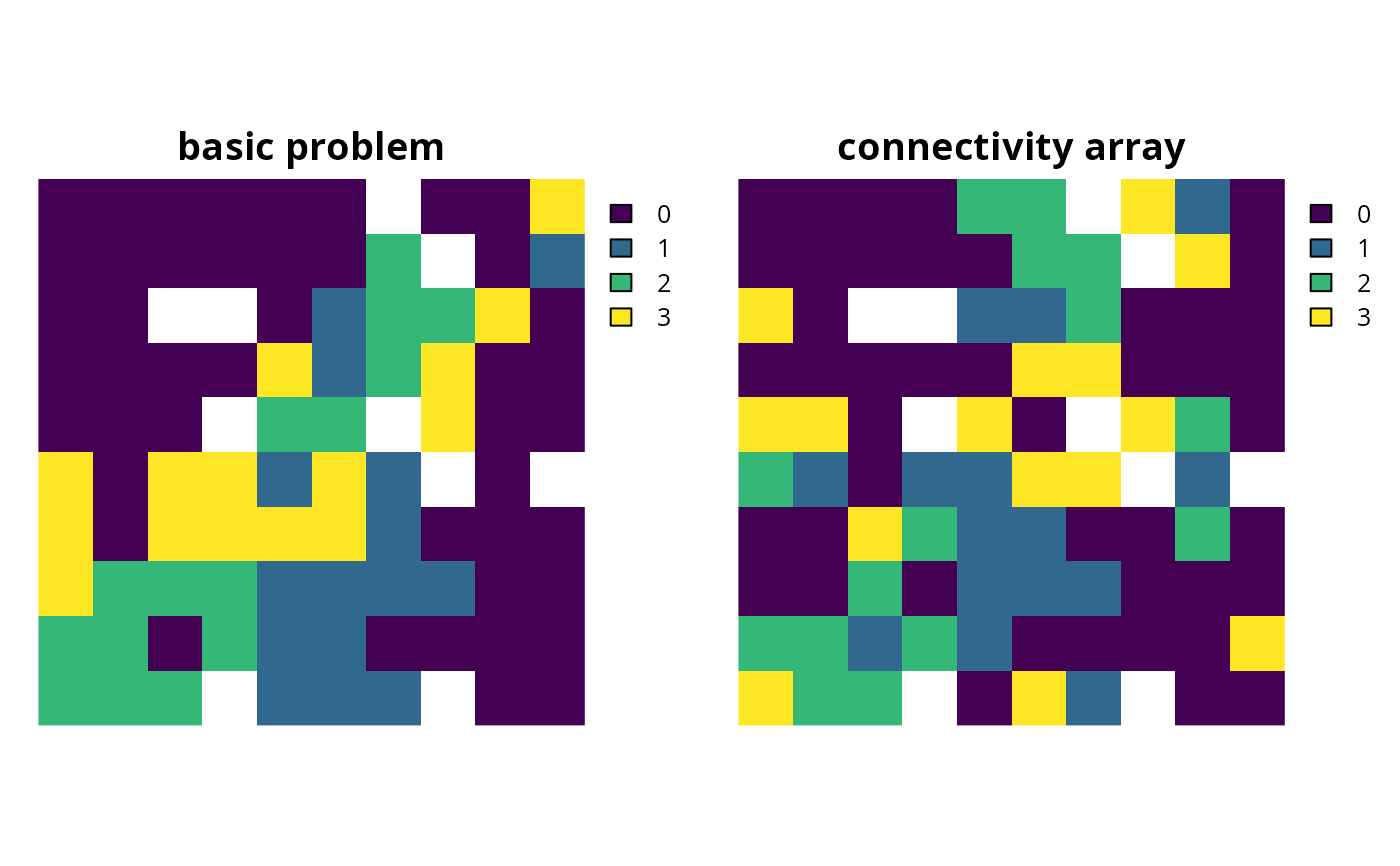# }